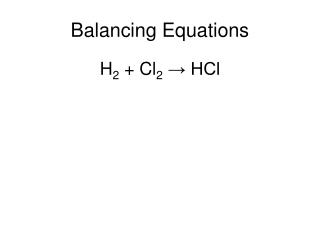DownloadDownload PresentationBalancing Equations

# Balancing Equations

Télécharger la présentation## Balancing Equations

- - - - - - - - - - - - - - - - - - - - - - - - - - - E N D - - - - - - - - - - - - - - - - - - - - - - - - - - -
##### Presentation Transcript

1. Balancing Equations H2 + Cl2→ HCl

2. Balancing Equations KI + Br2→ KBr + I2

3. Balancing Equations CH4 + O2→ CO2 + H2O

4. Balancing Equations V2O5 + Ca → V + CaO

5. Balancing Equations NaCl + F2 → NaF + Cl2

6. Using Mole Ratios In the reaction, 2H2O → 2H2 + O2, if you start with 2 mol of water, how many moles of hydrogen gas are produced?

7. Using Mole Ratios If you start with 5 mol of O2 in the reaction, 2Mg + O2→ 2MgO, how many moles of Mg will you need?

8. Using Mole Ratios In the reaction, H2S + 2O2→ H2SO4, the law of definite proportions predicts that for every mole of H2S you will need how many moles of O2?